# Finite Fields RongJaye Chen Finite fields n 1

• Slides: 17Finite Fields Rong-Jaye Chen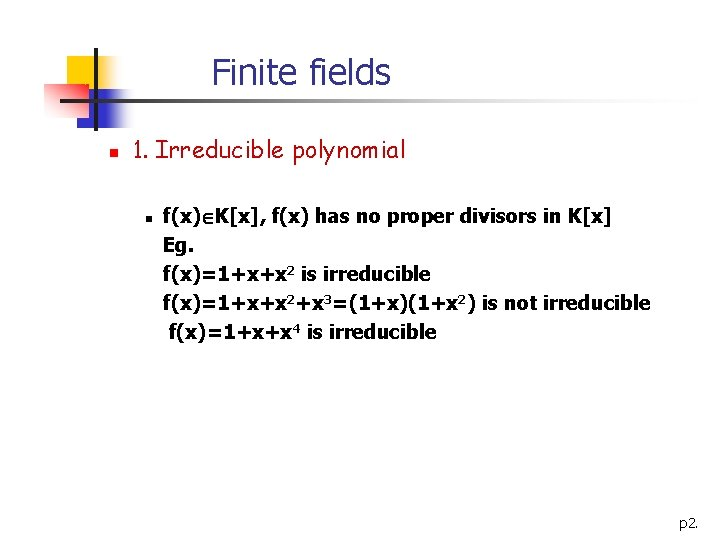Finite fields n 1. Irreducible polynomial n f(x) K[x], f(x) has no proper divisors in K[x] Eg. f(x)=1+x+x 2 is irreducible f(x)=1+x+x 2+x 3=(1+x)(1+x 2) is not irreducible f(x)=1+x+x 4 is irreducible p 2.Finite fields n 2. Primitive polynomial n n f(x) is irreducible of degree n > 1 f(x) is not a divisor of 1+xm for any m < 2 n-1 Eg. f(x)=1+x+x 2 is not a factor of 1+xm for m < 3 so f(x) is a primitive polynomial f(x)= 1+x+x 2+x 3+x 4 is irreducible but 1+x 5=(1+x)(1+x+x 2+x 3+x 4) and m=5 < 24 -1=15 so f(x) is not a primitive polynomial p 3.Finite fields n 3. Definition of Kn[x] n n The set of all polynomials in K[x] having degree less than n Each word in Kn corresponds to a polynomial in Kn[x] Multiplication in Kn modulo h(x), with irreducible h(x) of degree n If we use multiplication modulo a reducible h(x), say, 1+x 4 to define multiplication of words in K 4, however: (0101) (x+x 3) = x 2+x 6 = x 2+x 2 (mod 1+x 4) = 0 0000 (K 4 -{0000} is not closed under multiplication. ) p 4.Finite fields n Furthermore each nonzero element in Kn can have an inverse if we use irreducible h(x). But if we use reducible h(x) then there exists nonzero element, which has no inverse. Why? Let f(x) is nonzero element and h(x) is irreducible then gcd(f(x), h(x))=1 and so exists a(x)f(x)+b(x)h(x)=1 => a(x)f(x)=1 mod h(x) and so a(x) is the inverse of f(x) p 5.Finite fields n 4. Definition of Field (Kn, +, x) n n (K , +) is an abelian group with identity denoted 0 n The operation x is associative n a x ( b x c) = ( a x b ) x c n There is a multiplicative identity denoted 1, with 1 0 n n 1 x a = a x 1 = a, a K n The operation x is distributive over + n a x ( b + c ) = ( a x b ) + ( a x c ) n It is communicative n n a x b = b x a, b K n All non-zero elements have multiplicative inverses r n Galois Fields: GF(2 ) m n For every prime power order p , there is a unique finite field of order pm m n Denoted by GF(p ) p 6.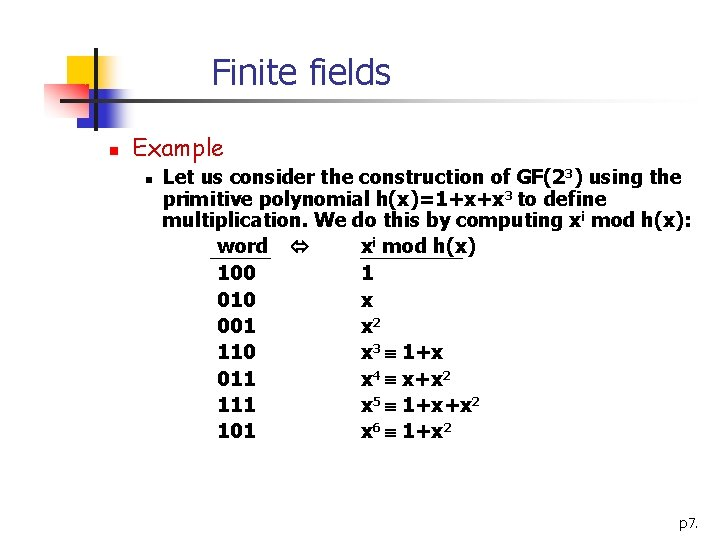Finite fields n Example n Let us consider the construction of GF(23) using the primitive polynomial h(x)=1+x+x 3 to define multiplication. We do this by computing xi mod h(x): word xi mod h(x) 100 1 010 x 001 x 2 110 x 3 1+x 011 x 4 x+x 2 111 x 5 1+x+x 2 101 x 6 1+x 2 p 7.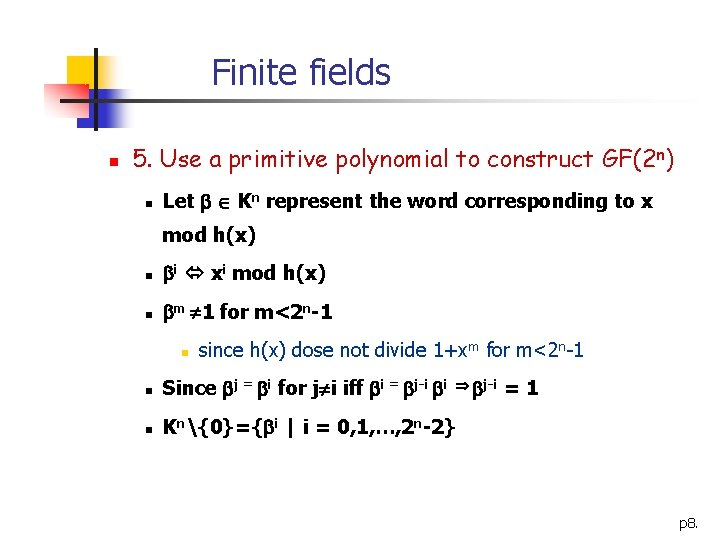Finite fields n 5. Use a primitive polynomial to construct GF(2 n) n Let Kn represent the word corresponding to x mod h(x) n i xi mod h(x) n m 1 for m<2 n-1 n since h(x) dose not divide 1+xm for m<2 n-1 n Since j = i for j i iff i = j-i i j-i n Kn{0}={ i | i = 0, 1, …, 2 n-2} =1 p 8.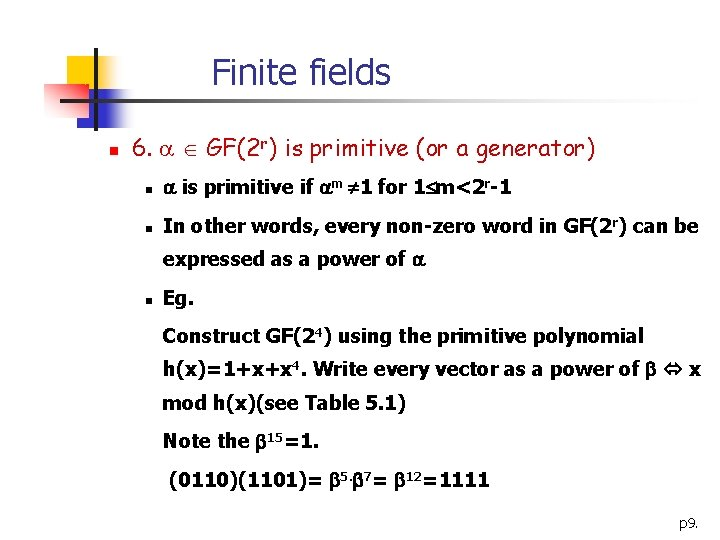Finite fields n 6. GF(2 r) is primitive (or a generator) n is primitive if m 1 for 1 m<2 r-1 n In other words, every non-zero word in GF(2 r) can be expressed as a power of n Eg. Construct GF(24) using the primitive polynomial h(x)=1+x+x 4. Write every vector as a power of x mod h(x)(see Table 5. 1) Note the 15=1. (0110)(1101)= 5. 7= 12=1111 p 9.Minimal polynomials § 1. Root of a polynomial § : an element of F=GF(2 r), p(x) F[x] § is a root of a polynomial p(x) iff p( )=0 § 2. Order of § The smallest positive integer m such that m=1 § in GF(2 r) is a primitive element if it has order 2 r-1 p 10.Minimal polynomials § 3. Minimal polynomial of § The polynomial in K[x] of smallest degree having as root § Denoted by m (x) § m (x) is irreducible over K § If f(x) is any polynomial over K such that f( )=0, then m (x) is a factor of f(x) § m (x) is unique § m (x) is a factor of p 11.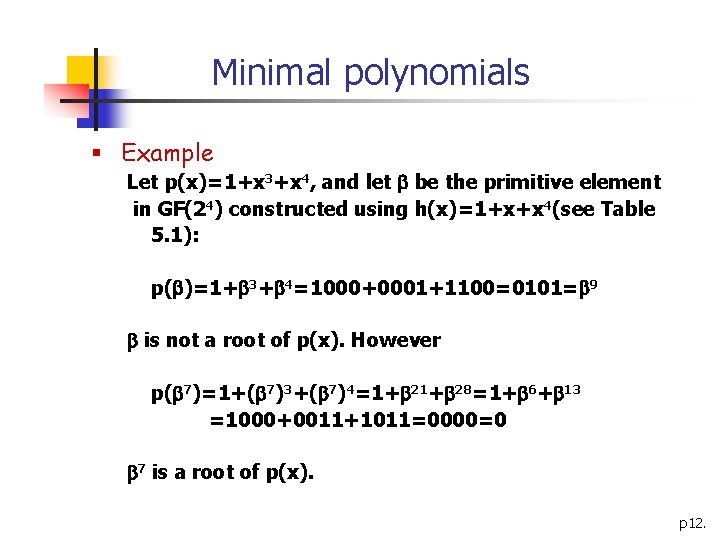Minimal polynomials § Example Let p(x)=1+x 3+x 4, and let be the primitive element in GF(24) constructed using h(x)=1+x+x 4(see Table 5. 1): p( )=1+ 3+ 4=1000+0001+1100=0101= 9 is not a root of p(x). However p( 7)=1+( 7)3+( 7)4=1+ 28=1+ 6+ 13 =1000+0011+1011=0000=0 7 is a root of p(x). p 12.Minimal polynomials § 4. Finding the minimal polynomial of § Reduce to find a linear combination of the vectors{1, , 2, …, r}, which sums to 0 § Any set of r+1 vectors in Kr is dependent, such a solution exists § Represent m (x) by mi(x) where = i § eg. Find the m (x), = 3, GF(24) constructed using h(x)=1+x+x 4 p 13.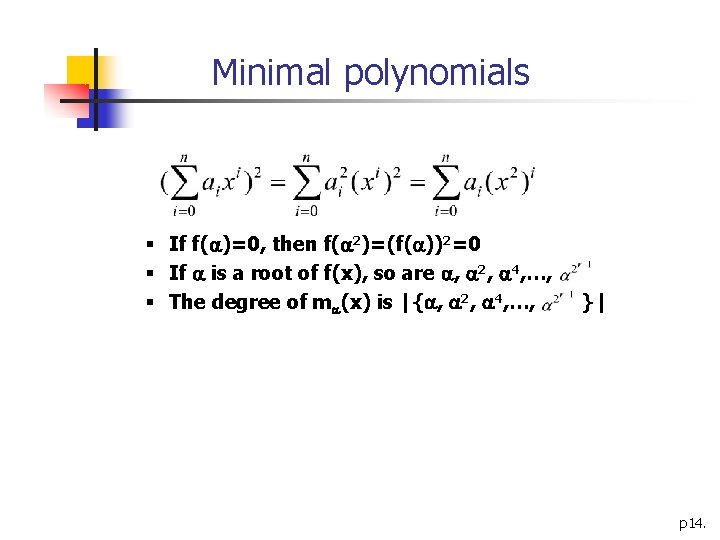Minimal polynomials § If f( )=0, then f( 2)=(f( ))2=0 § If is a root of f(x), so are , 2, 4, …, § The degree of m (x) is |{ , 2, 4, …, }| p 14.Minimal polynomials § Example § Find the m (x), = 3, GF(24) constructed using h(x)=1+x+x 4 § Let m (x)= m 3(x)=a 0+a 1 x+a 2 x 2+a 3 x 3+a 4 x 4 then we must find the value for a 0, a 1, …, a 4 {0, 1} m ( )=0=a 01+a 1 +a 2 2+a 3 3+a 4 4 =a 0 0+a 1 3+a 2 6+a 3 9+a 4 12 0000=a 0(1000)+a 1(0001)+a 2(0011)+a 3(0101)+ a 4(1111) a 0=a 1=a 2=a 3=a 4=1 and m (x)=1+x+x 2+x 3+x 4 p 15.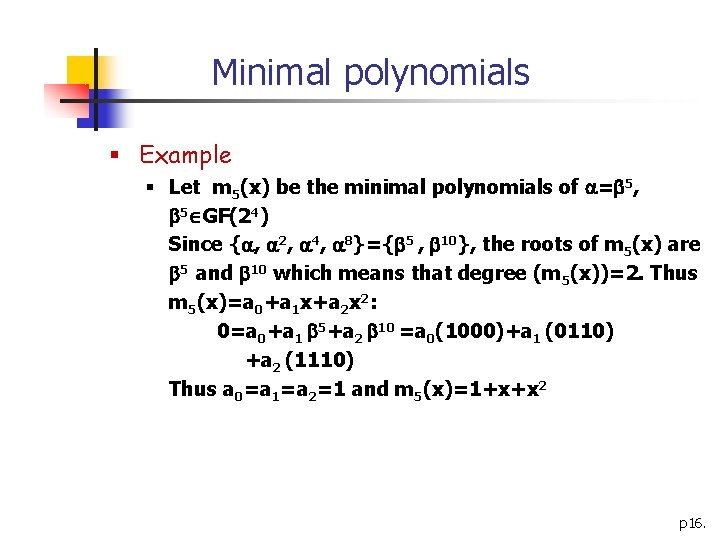Minimal polynomials § Example § Let m 5(x) be the minimal polynomials of = 5, 5 GF(24) Since { , 2, 4, 8}={ 5 , 10}, the roots of m 5(x) are 5 and 10 which means that degree (m 5(x))=2. Thus m 5(x)=a 0+a 1 x+a 2 x 2: 0=a 0+a 1 5+a 2 10 =a 0(1000)+a 1 (0110) +a 2 (1110) Thus a 0=a 1=a 2=1 and m 5(x)=1+x+x 2 p 16.Minimal polynomials § Table 5. 2: Minimal polynomials in GF(24) constructed using 1+x+x 4 Element of GF(24) 0 1 , 2, 4, 8 3, 6, 9, 12 5, 10 7, 11, 13, 14 Minimal polynomial x 1+x+x 4 1+x+x 2+x 3+x 4 1+x+x 2 1+x 3+x 4 p 17.